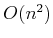# 6.5 Complexity of Motion Planning

This section summarizes theoretical work that characterizes the complexity of motion planning problems. Note that this is not equivalent to characterizing the running time of particular algorithms. The existence of an algorithm serves as an upper bound on the problem's difficulty because it is a proof by example that solving the problem requires no more time than what is needed by the algorithm. On the other hand, lower bounds are also very useful because they give an indication of the difficulty of the problem itself. Suppose, for example, you are given an algorithm that solves a problem in time. Does it make sense to try to find a more efficient algorithm? Does it make sense to try to find a general-purpose motion planning algorithm that runs in time that is polynomial in the dimension? Lower bounds provide answers to questions such as this. Usually lower bounds are obtained by concocting bizarre, complicated examples that are allowed by the problem definition but were usually not considered by the person who first formulated the problem. In this line of research, progress is made by either raising the lower bound (unless it is already tight) or by showing that a narrower version of the problem still allows such bizarre examples. The latter case occurs often in motion planning.

Subsections
Steven M LaValle 2020-08-14